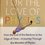# Combinatorics!

Hi, how do you hunt for the number of solutions of :

$$0 \leq a_{1} \leq a_{2} \leq a_{3} \dots \leq a_{m} \leq a$$.

Here is the solution:

Let $S =$ {$a_{1}, a_{2}, \dots a_{m}$},

Clearly, if we find this set, afterwards, there is only $1$ way of distribution, since the order is fixed.

Say, amongst the set $S$, the integer $r$ comes $P_{r}$ times, then:

$\displaystyle \sum_{r=0}^{a} P_{r} = m$, and $P_{r} \geq 0$

We know that the number of the solutions of this typical equation is ${m+a \choose a}$, hence , the set is chosen, and hence the required number of solutions of the original equation is also ${m+a \choose a }$.

_Below is an interesting problem: _

We want to create a Divisible Sequence of length $H$ from a number $N$. In a Divisible Sequence, every term (except the starting number) is a divisor of the previous term. Examples of Divisible Sequences of length $3$ starting with $10$ are:

$10,10,10$

$10, 10, 5$

$10, 2, 2$

$10, 10, 1$

$10, 1, 1$

For primes $p_{1},p_{2}, \dots p_{t}$, obtain an expression for the number of divisible sequences starting with $p_{1}^{q_{1}} p_{2}^{q_{2}} \dots p_{t}^{q_{t}}$, of length $k+1$.

$0 \leq q_{i} \forall i$

Note: You might leave this expression in a sum or a product form.7 years, 4 months ago

This discussion board is a place to discuss our Daily Challenges and the math and science related to those challenges. Explanations are more than just a solution — they should explain the steps and thinking strategies that you used to obtain the solution. Comments should further the discussion of math and science.

When posting on Brilliant:

• Use the emojis to react to an explanation, whether you're congratulating a job well done , or just really confused .
• Ask specific questions about the challenge or the steps in somebody's explanation. Well-posed questions can add a lot to the discussion, but posting "I don't understand!" doesn't help anyone.
• Try to contribute something new to the discussion, whether it is an extension, generalization or other idea related to the challenge.

MarkdownAppears as
*italics* or _italics_ italics
**bold** or __bold__ bold
- bulleted- list
• bulleted
• list
1. numbered2. list
1. numbered
2. list
Note: you must add a full line of space before and after lists for them to show up correctly
paragraph 1paragraph 2

paragraph 1

paragraph 2

[example link](https://brilliant.org)example link
> This is a quote
This is a quote
    # I indented these lines
# 4 spaces, and now they show
# up as a code block.

print "hello world"
# I indented these lines
# 4 spaces, and now they show
# up as a code block.

print "hello world"
MathAppears as
Remember to wrap math in $$ ... $$ or $ ... $ to ensure proper formatting.
2 \times 3 $2 \times 3$
2^{34} $2^{34}$
a_{i-1} $a_{i-1}$
\frac{2}{3} $\frac{2}{3}$
\sqrt{2} $\sqrt{2}$
\sum_{i=1}^3 $\sum_{i=1}^3$
\sin \theta $\sin \theta$
\boxed{123} $\boxed{123}$

Sort by:

can u explain it more easily ?

- 7 years, 3 months ago